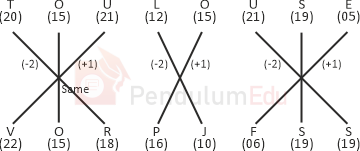Question of The Day19-07-2021

In a certain code language, ’PRUDENCE’ is written as ‘VRNFBFCL’. How will ‘TOULOUSE’ be written as in code language?

Correct Answer : a ) VOUPLFSS

Explanation :

According to the question

Let us assume A is numbered as 1, B as 2 and so on till Z being numbered as 26

Code for ’PRUDENCE’ is VRNFBFCL

The common logic applied here isSimilarly, the code for TOULOUSE isThus, the code for TOULOUSE is VOUPLFSS

Hence, (a) is the correct answer.0